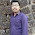## Monday, April 9, 2018

### Pandas groupby example

Pandas groupby function is really useful and powerful in many ways. This week, I am going to show some examples of using this groupby functions that I usually use in my analysis.
import pandas as pd
import matplotlib.pyplot as plt
import numpy as np
plt.style.use('seaborn-poster')

%matplotlib inline
Let's first create a DataFrame, that contains the name, score and round for some games.
a = ['Qingkai', 'Ironman', 'Batman', 'Qingkai', 'Ironman', 'Qingkai', 'Batman']
b = [3., 4, 2., 4, 5, 1, 2]
c = range(len(a))

d = [[x,y,z] for x,y,z in zip(a,b,c)]

df = pd.DataFrame(d, columns=['name', 'score', 'round'])
df
Now I want to calculate the mean scores for different users across all the games and the standard deviations. It could be quite simple with Pandas. As I am showing below:
df.groupby('name').mean()
scoreround
name
Batman2.0000004.000000
Ironman4.5000002.500000
Qingkai2.6666672.666667
df.groupby('name').std()
scoreround
name
Batman0.0000002.828427
Ironman0.7071072.121320
Qingkai1.5275252.516611
Or I can loop through the groupby object once to calculate them all.
for ix, grp in df.groupby('name'):
print('Name: %s, mean: %.1f, std: %.1f'%(ix, grp['score'].mean(), grp['score'].std()))
Name: Batman, mean: 2.0, std: 0.0
Name: Ironman, mean: 4.5, std: 0.7
Name: Qingkai, mean: 2.7, std: 1.5
But also, we could do it with one liner using the agg function:
df.groupby('name').agg({'score':['mean','std'],'round':'count'})
scoreround
meanstdcount
name
Batman2.0000000.0000002
Ironman4.5000000.7071072
Qingkai2.6666671.5275253
Besides, you can also use some customized functions in the agg as well. For example, if we want to calculate the RMS value of the score, we could do the following:
def cal_RMS(x):
return np.sqrt(sum(x**2/len(x)))
df.groupby('name').agg({'score':['mean',cal_RMS],'round':'count'})
scoreround
meancal_RMScount
name
Batman2.0000002.0000002
Ironman4.5000004.5276932
Qingkai2.6666672.9439203

1.2.3.4.